# 深度学习之卷积神经网络CNN及tensorflow代码实现示例

## 一、CNN的引入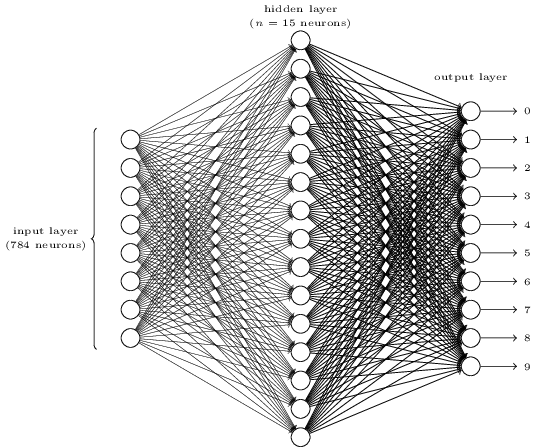• 输入层：用于数据的输入
• 卷积层：使用卷积核进行特征提取和特征映射
• 激励层：由于卷积也是一种线性运算，因此需要增加非线性映射
• 池化层：进行下采样，对特征图稀疏处理，减少数据运算量。
• 全连接层：通常在CNN的尾部进行重新拟合，减少特征信息的损失
• 输出层：用于输出结果

• 归一化层（Batch Normalization）：在CNN中对特征的归一化
• 切分层：对某些（图片）数据的进行分区域的单独学习
• 融合层：对独立进行特征学习的分支进行融合

## 二、CNN的层次结构

### 输入层：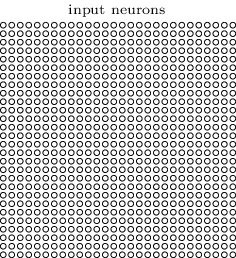### 卷积层：

• local receptive fields（感受视野）
• shared weights（共享权值）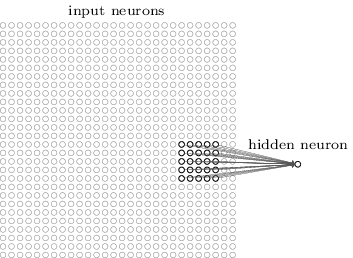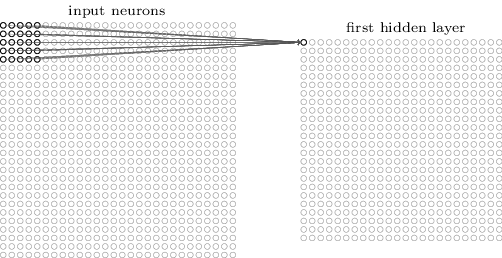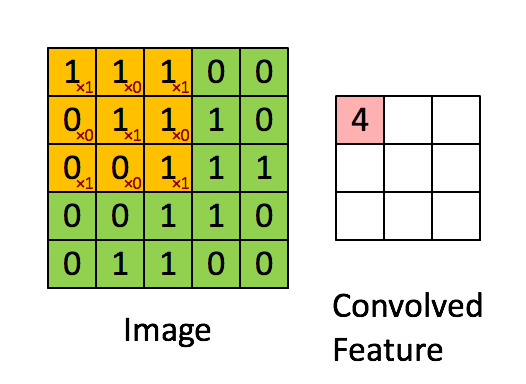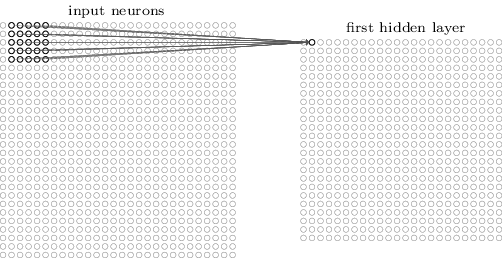b+i=04j=04wijxij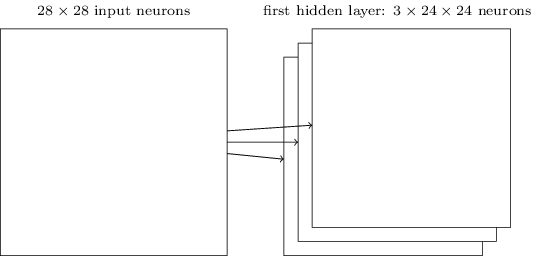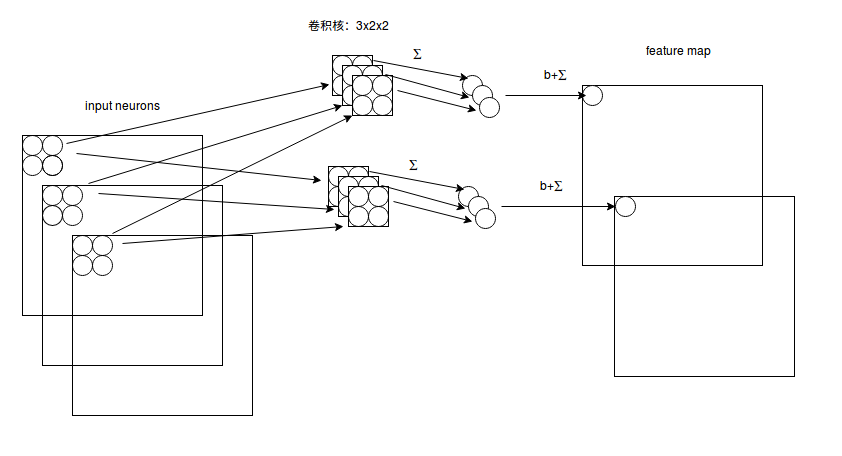f(x)=max(x,0)

### 池化层：

• Max pooling：取“池化视野”矩阵中的最大值
• Average pooling：取“池化视野”矩阵中的平均值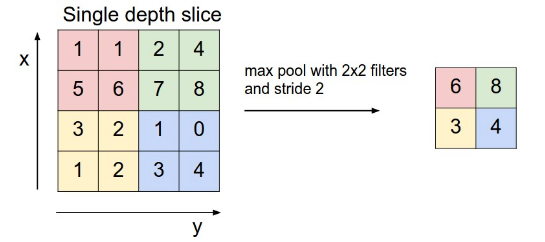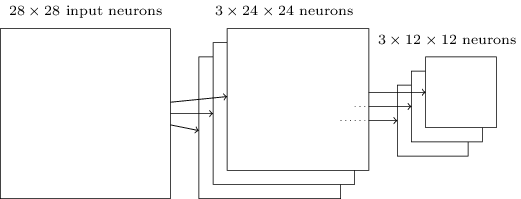### 归一化层：

#### 1. Batch Normalization

Batch Normalization（批量归一化）实现了在神经网络层的中间进行预处理的操作，即在上一层的输入归一化处理后再进入网络的下一层，这样可有效地防止“梯度弥散”，加速网络训练。

Batch Normalization具体的算法如下图所示：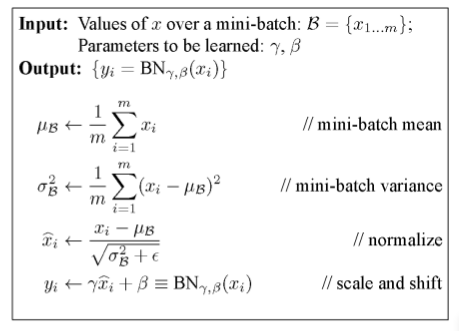Batch Normalization 算法的训练过程和测试过程的区别：

#### 2. Local Response Normalization

LRN的公式如下：

b(i,x,y)=a(i,x,y)(k+αmin(N1,i+n/2)j=max(0,in/2)a(j,x,y)2) β

a(i,x,y)$a(i,x,y)$ 表示第i$i$个卷积核的输出（经过ReLu层）的feature map上的 (xy)$(x，y )$ 位置上的值。
b(i,x,y)$b(i,x,y)$ 表示 a(i,x,y)$a(i,x,y)$ 经LRN后的输出。
N$N$ 表示卷积核的数量，即输入的 feature map的个数。
n$n$ 表示近邻的卷积核（或feature map）个数，由自己来决定。
k,α,β$k,\alpha,\beta$是超参数，由用户自己调整或决定。

### 融合层：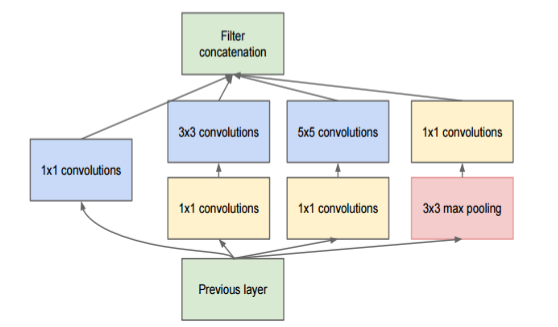### 全连接层和输出层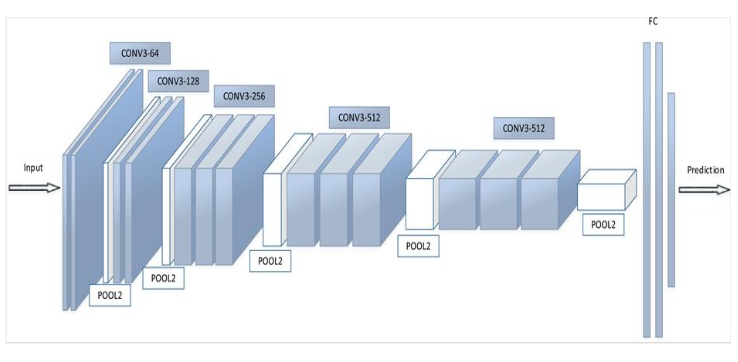### 典型的卷积神经网络

#### LeNet-5模型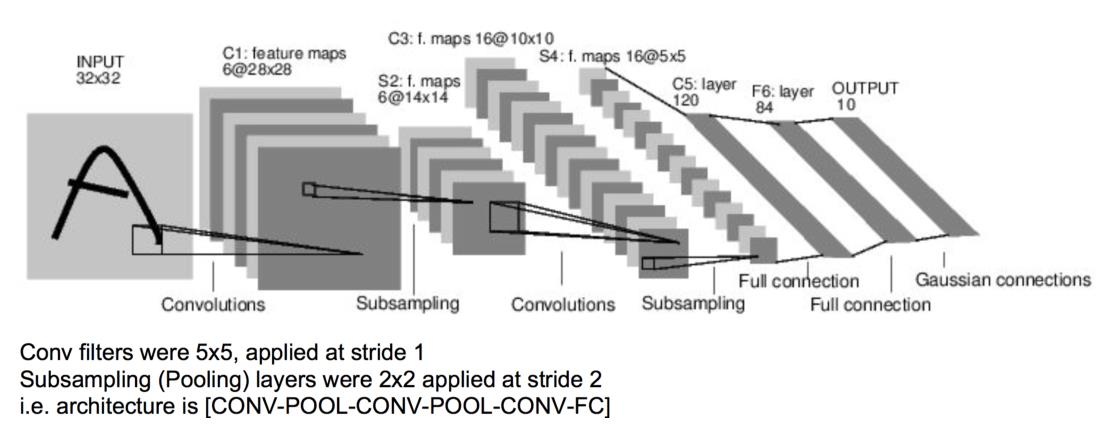#### AlexNet 模型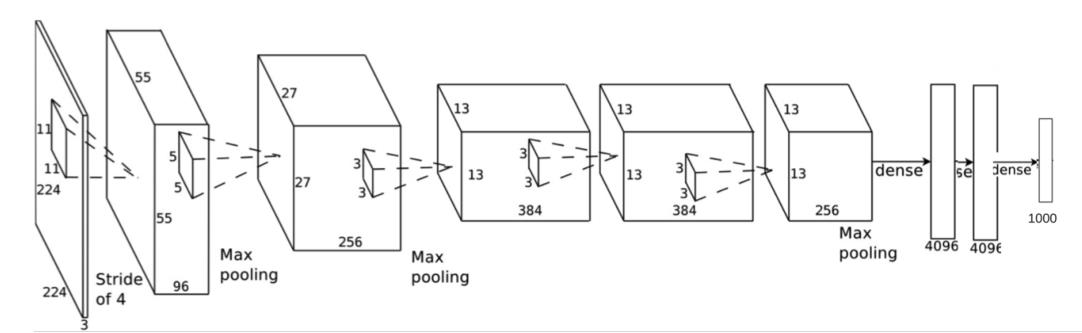(+)++

AlexNet卷积层的卷积核边长为5或3，池化层的窗口边长为3。具体参数如图所示：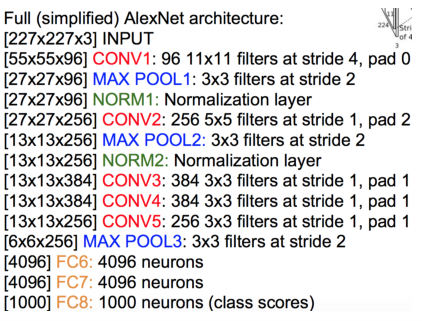#### VGGNet 模型

VGGNet 模型 和 AlexNet模型 在结构上没多大变化，在卷积层部位增加了多个卷积层。AlexNet（上） 和 VGGNet （下）的对比如下图所示：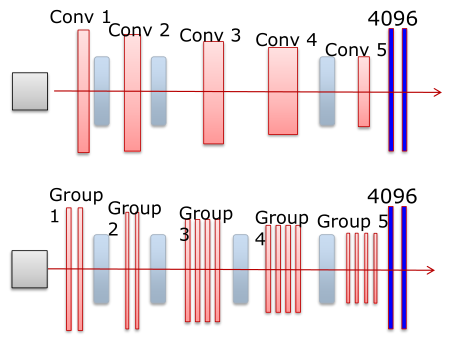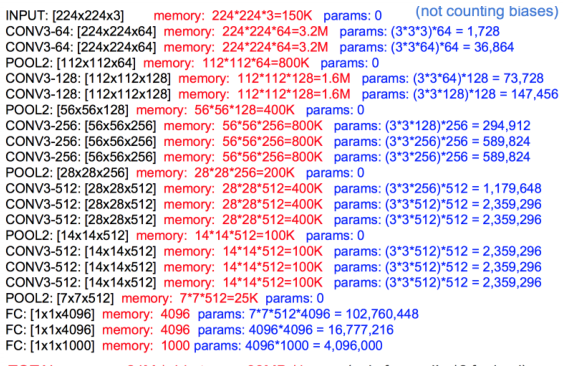#### GoogleNet 模型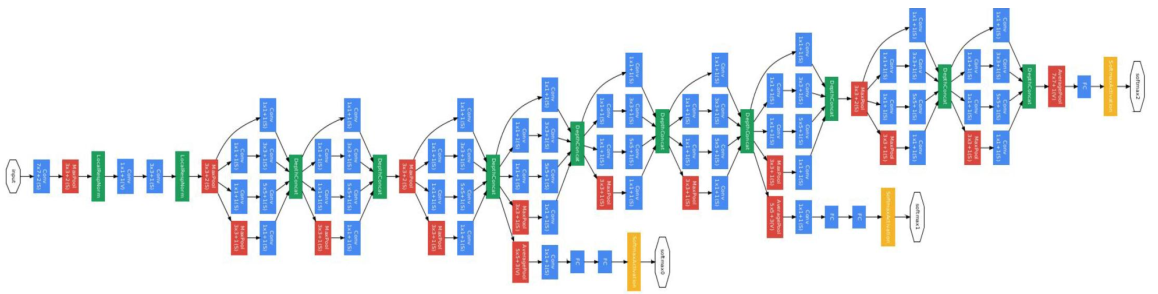GoogleNet还有一个特点就是它是全卷积结构（FCN）的，网络的最后没有使用全连接层，一方面这样可以减少参数的数目，不容易过拟合，一方面也带来了一些空间信息的丢失。代替全连接层的是全局平均池化（Global Average Pooling，GAP）的方法，思想是：为每一个类别输出一个 feature map ，再取每一个 feature map上的平均值，作为最后的softmax层的输入。

#### ResNet模型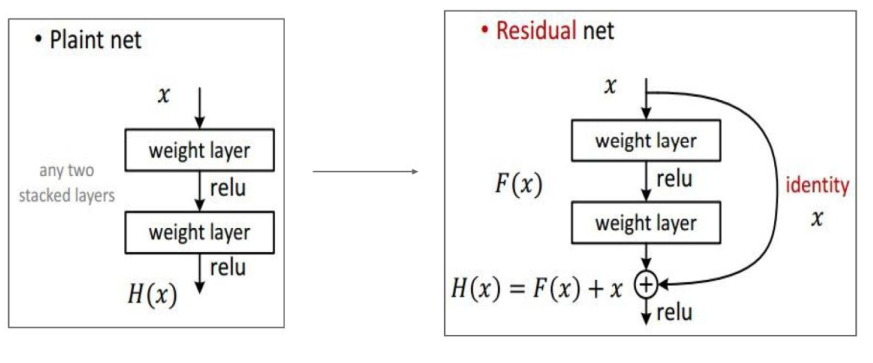### 四、Tensorflow代码

#### 主要的函数说明：

tf.nn.conv2d(input, filter, strides, padding, use_cudnn_on_gpu=None, data_format=None, name=None)

##### 参数说明：
• data_format：表示输入的格式，有两种分别为：“NHWC”和“NCHW”，默认为“NHWC”

• input：输入是一个4维格式的（图像）数据，数据的 shape 由 data_format 决定：当 data_format 为“NHWC”输入数据的shape表示为[batch, in_height, in_width, in_channels]，分别表示训练时一个batch的图片数量、图片高度、 图片宽度、 图像通道数。当 data_format 为“NHWC”输入数据的shape表示为[batch, in_channels， in_height, in_width]

• filter：卷积核是一个4维格式的数据：shape表示为：[height,width,in_channels, out_channels]，分别表示卷积核的高、宽、深度（与输入的in_channels应相同）、输出 feature map的个数（即卷积核的个数）。

• strides：表示步长：一个长度为4的一维列表，每个元素跟data_format互相对应，表示在data_format每一维上的移动步长。当输入的默认格式为：“NHWC”，则 strides = [batch , in_height , in_width, in_channels]。其中 batch 和 in_channels 要求一定为1，即只能在一个样本的一个通道上的特征图上进行移动，in_height , in_width表示卷积核在特征图的高度和宽度上移动的布长，即 strideheight$stride_{height}$stridewidth$stride_{width}$

• padding：表示填充方式：“SAME”表示采用填充的方式，简单地理解为以0填充边缘，当stride为1时，输入和输出的维度相同；“VALID”表示采用不填充的方式，多余地进行丢弃。具体公式：

“SAME”: output_spatial_shape[i]=(input_spatial_shape[i] / strides[i])$output\_spatial\_shape[i] = \lceil (input\_spatial\_shape[i]\ /\ strides[i] )\rceil$

“VALID”: output_spatial_shape[i]=((input_spatial_shape[i](spatial_filter_shape[i]1)/strides[i])$output\_spatial\_shape[i] = \lceil ((input\_spatial\_shape[i] - (spatial\_filter\_shape[i]-1) / strides[i])\rceil$

tf.nn.max_pool( value, ksize,strides,padding,data_format=’NHWC’,name=None)

tf.nn.avg_pool(…)

##### 参数说明：
• value：表示池化的输入：一个4维格式的数据，数据的 shape 由 data_format 决定，默认情况下shape 为[batch, height, width, channels]

• 其他参数与 tf.nn.cov2d 类型

• ksize：表示池化窗口的大小：一个长度为4的一维列表，一般为[1, height, width, 1]，因不想在batch和channels上做池化，则将其值设为1。

Batch Nomalization层：
batch_normalization( x,mean,variance,offset,scale, variance_epsilon,name=None)

• mean 和 variance 通过 tf.nn.moments 来进行计算：
batch_mean, batch_var = tf.nn.moments(x, axes = [0, 1, 2], keep_dims=True)，注意axes的输入。对于以feature map 为维度的全局归一化，若feature map 的shape 为[batch, height, width, depth]，则将axes赋值为[0, 1, 2]

• x 为输入的feature map 四维数据，offset、scale为一维Tensor数据，shape 等于 feature map 的深度depth。

#### 代码示例：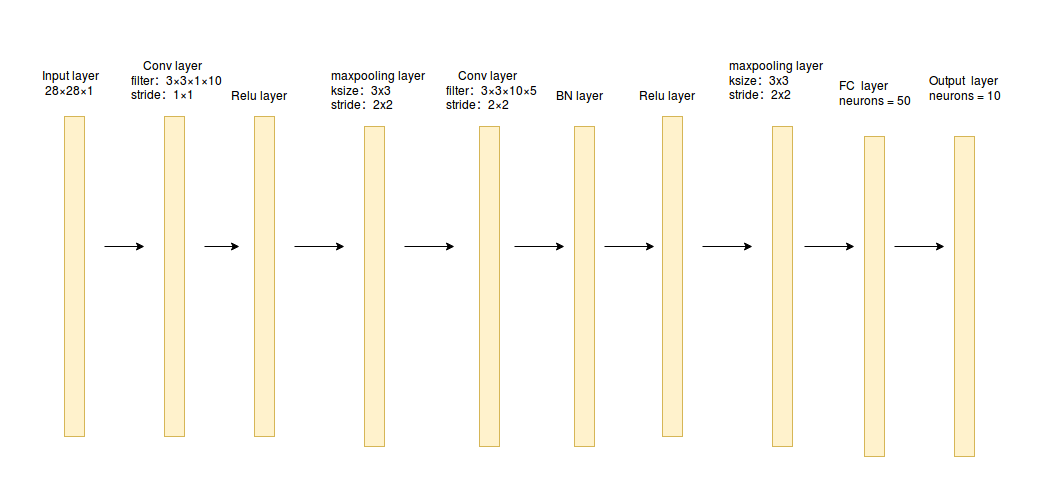import tensorflow as tf
from sklearn.datasets import load_digits
import numpy as np
digits = load_digits()
X_data = digits.data.astype(np.float32)
Y_data = digits.target.astype(np.float32).reshape(-1,1)
print X_data.shape
print Y_data.shape
(1797, 64)
(1797, 1)

from sklearn.preprocessing import MinMaxScaler
scaler = MinMaxScaler()
X_data = scaler.fit_transform(X_data)
from sklearn.preprocessing import OneHotEncoder
Y = OneHotEncoder().fit_transform(Y_data).todense() #one-hot编码
Y
matrix([[ 1.,  0.,  0., ...,  0.,  0.,  0.],
[ 0.,  1.,  0., ...,  0.,  0.,  0.],
[ 0.,  0.,  1., ...,  0.,  0.,  0.],
...,
[ 0.,  0.,  0., ...,  0.,  1.,  0.],
[ 0.,  0.,  0., ...,  0.,  0.,  1.],
[ 0.,  0.,  0., ...,  0.,  1.,  0.]])

# 转换为图片的格式 （batch，height，width，channels）
X = X_data.reshape(-1,8,8,1)
batch_size = 8 # 使用MBGD算法，设定batch_size为8
def generatebatch(X,Y,n_examples, batch_size):
for batch_i in range(n_examples // batch_size):
start = batch_i*batch_size
end = start + batch_size
batch_xs = X[start:end]
batch_ys = Y[start:end]
yield batch_xs, batch_ys # 生成每一个batch
tf.reset_default_graph()
# 输入层
tf_X = tf.placeholder(tf.float32,[None,8,8,1])
tf_Y = tf.placeholder(tf.float32,[None,10])
# 卷积层+激活层
conv_filter_w1 = tf.Variable(tf.random_normal([3, 3, 1, 10]))
conv_filter_b1 =  tf.Variable(tf.random_normal())
relu_feature_maps1 = tf.nn.relu(\
tf.nn.conv2d(tf_X, conv_filter_w1,strides=[1, 1, 1, 1], padding='SAME') + conv_filter_b1)
# 池化层
max_pool1 = tf.nn.max_pool(relu_feature_maps1,ksize=[1,3,3,1],strides=[1,2,2,1],padding='SAME')
print max_pool1
Tensor("MaxPool:0", shape=(?, 4, 4, 10), dtype=float32)

# 卷积层
conv_filter_w2 = tf.Variable(tf.random_normal([3, 3, 10, 5]))
conv_filter_b2 =  tf.Variable(tf.random_normal())
conv_out2 = tf.nn.conv2d(relu_feature_maps1, conv_filter_w2,strides=[1, 2, 2, 1], padding='SAME') + conv_filter_b2
print conv_out2
Tensor("add_4:0", shape=(?, 4, 4, 5), dtype=float32)

# BN归一化层+激活层
batch_mean, batch_var = tf.nn.moments(conv_out2, [0, 1, 2], keep_dims=True)
shift = tf.Variable(tf.zeros())
scale = tf.Variable(tf.ones())
epsilon = 1e-3
BN_out = tf.nn.batch_normalization(conv_out2, batch_mean, batch_var, shift, scale, epsilon)
print BN_out
relu_BN_maps2 = tf.nn.relu(BN_out)
Tensor("batchnorm/add_1:0", shape=(?, 4, 4, 5), dtype=float32)

# 池化层
max_pool2 = tf.nn.max_pool(relu_BN_maps2,ksize=[1,3,3,1],strides=[1,2,2,1],padding='SAME')
print max_pool2
Tensor("MaxPool_1:0", shape=(?, 2, 2, 5), dtype=float32)

# 将特征图进行展开
max_pool2_flat = tf.reshape(max_pool2, [-1, 2*2*5])
# 全连接层
fc_w1 = tf.Variable(tf.random_normal([2*2*5,50]))
fc_b1 =  tf.Variable(tf.random_normal())
fc_out1 = tf.nn.relu(tf.matmul(max_pool2_flat, fc_w1) + fc_b1)
# 输出层
out_w1 = tf.Variable(tf.random_normal([50,10]))
out_b1 = tf.Variable(tf.random_normal())
pred = tf.nn.softmax(tf.matmul(fc_out1,out_w1)+out_b1)
loss = -tf.reduce_mean(tf_Y*tf.log(tf.clip_by_value(pred,1e-11,1.0)))
train_step = tf.train.AdamOptimizer(1e-3).minimize(loss)
y_pred = tf.arg_max(pred,1)
bool_pred = tf.equal(tf.arg_max(tf_Y,1),y_pred)
accuracy = tf.reduce_mean(tf.cast(bool_pred,tf.float32)) # 准确率
with tf.Session() as sess:
sess.run(tf.global_variables_initializer())
for epoch in range(1000): # 迭代1000个周期
for batch_xs,batch_ys in generatebatch(X,Y,Y.shape,batch_size): # 每个周期进行MBGD算法
sess.run(train_step,feed_dict={tf_X:batch_xs,tf_Y:batch_ys})
if(epoch%100==0):
res = sess.run(accuracy,feed_dict={tf_X:X,tf_Y:Y})
print (epoch,res)
res_ypred = y_pred.eval(feed_dict={tf_X:X,tf_Y:Y}).flatten() # 只能预测一批样本，不能预测一个样本
print res_ypred
(0, 0.36338341)
(100, 0.96828049)
(200, 0.99666113)
(300, 0.99554813)
(400, 0.99888706)
(500, 0.99777406)
(600, 0.9961046)
(700, 0.99666113)
(800, 0.99499166)
(900, 0.99888706)
[0 1 2 ..., 8 9 8]


from sklearn.metrics import  accuracy_score
print accuracy_score(Y_data,res_ypred.reshape(-1,1))
0.998887033945